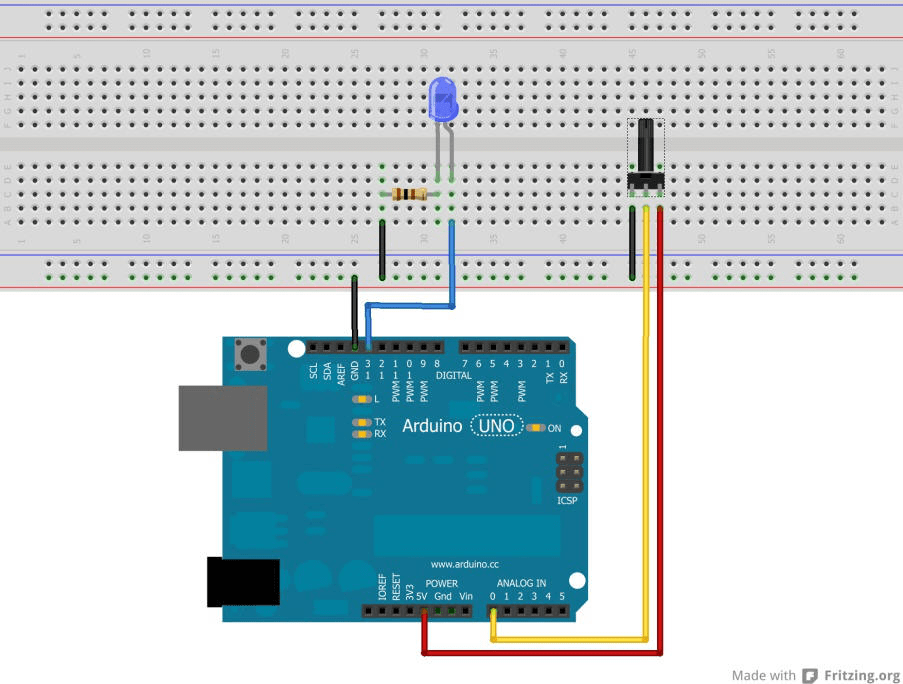# No. 9 – Potentiometer

Task: The speed of a blinking LED should be regulated with a potentiometer.

Required equipment: Arduino / Potentiometer / Breadboard / Cables

Learning content: Reading out a potentiometer and working with sensor data on a mathematical basis (in this case for the duration of a break).

There are three contacts on a potentiometer. The outer ones should be connected with + and –. The contact in the middle has to be connected with a analog Input on the microcontroller. If the potentiometer gets turned around, the pin in the middle will give out a voltage between 0V and 5V. Potentiometer on the most left position: 0V and potentiometer on the most right position: 5V, depending on the way it’s connected (+ and -).

We will use the already fixed LED with pin 13 on the microcontroller. But it’s also possible to connect an additional LED on the Breadboard like it’s shown here:Code:

int input=A0; //The word “input” now stands for the value “A0”

int LED=13; //”LED” now stand for the value 13

int sensorvalue=0; //Variable for the sensorvalue with 0 as starting value

void setup()

{ //The setup begins here

pinMode (LED,OUTPUT); //The pin 13 connected to the LED is defined as an output

}

void loop()

{ //The loop part begins here

sensorvalue= analogRead(input); //The voltage at the potentiometer is read out and gets saved as a number //between 0 and 1023 under “sensorvalue”

digitalWrite (LED,HIGH); //Turn on LED

delay(sensorvalue); //The value, which is saved under “sensorvalue” defines how long the LED will light up //(milliseconds)

digitalWrite(LED, LOW); //Turn off LED

delay(sensorvalue); //The value, which is saved under “sensorvalue” defines how long the LED is turned off //(milliseconds)

} //End of the loop part

//Now the loop part will be restarted. If the read out value of the potentiometer has changed, the time //between the on and off stage will also change. The LED will be blinking faster or slower. The longest //possible delay in this sketch can be 1023ms (milliseconds) long. If needed longer delays are also //possible. Therefore you would have to add a litte mathimatical function into the sketch. Example: The //line “sensorvalue=analogRead(input);” has to be changed into “sensorvalue=analogRead(input)*2;”. The //saved sensor value will be increased by the factor of 2. So the longest delay would be 2046ms and so on..